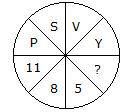# Verbal Reasoning - Character Puzzles - Discussion

### Discussion :: Character Puzzles - Character Puzzles 1 (Q.No.32)

32.

Which one will replace the question mark ?[A]. 3 [B]. 2 [C]. 7 [D]. 6

Explanation:

Putting the position of the letters in reverse order

P = 11, S = 8, V = 5 and Y = 2.

 Sherin said: (Dec 29, 2010) y is 2 selected i guess it should be 3 as difference between numbers is 3

 Munmi said: (Sep 19, 2016) The answer will b A.

 Bishen said: (Mar 15, 2019) @Sherin and @Munmi. The answer is not 3. Because 11-3=8, 8-3=5, 5-3=2. So, the answer is 2, as you can see there is a difference between these numbers is 3.

 Naveena said: (Oct 11, 2020) Here each letter and number difference is 3 in that way, y=2.